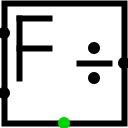#Floating Point Divider

 Library: Arithmetic Introduced: 3.5 Appearance:## Behavior

This component divides the dividend floating-point values (west edge - north end) by the divisor floating-point values (west edge - south end) and outputs the quotient floating-point values (East edge).
It has an error output in the south, which is set to 1 if the component receives an error(E) or undefined(U) signal or the floating-point values NaN

## Pins

West edge, north end:
Input: The floating-point values of the dividend. Bit width matches the Data Float size attribute.
West edge, south end:
Input: The floating-point values of the divisor. Bit width matches the Data Float size attribute.
East edge:
Output: The floating-point values of the quotient, the result of the division. Bit width matches the Data Float size attribute.
South edge:
OutPut: Error Set to 1 if the negator receives an error (E) or undefined (U) signal or the floating-point values NaN

## Attributes

When the component is selected or being added, Alt-0 throught Alt-9 alter its Float size attribute. Only 32 or 64bits

Float size
The bit width of the floating-point values to be divided and of the result. Only 32 or 64 bits

None.

## Text Tool Behavior

None.

Back to Library Reference